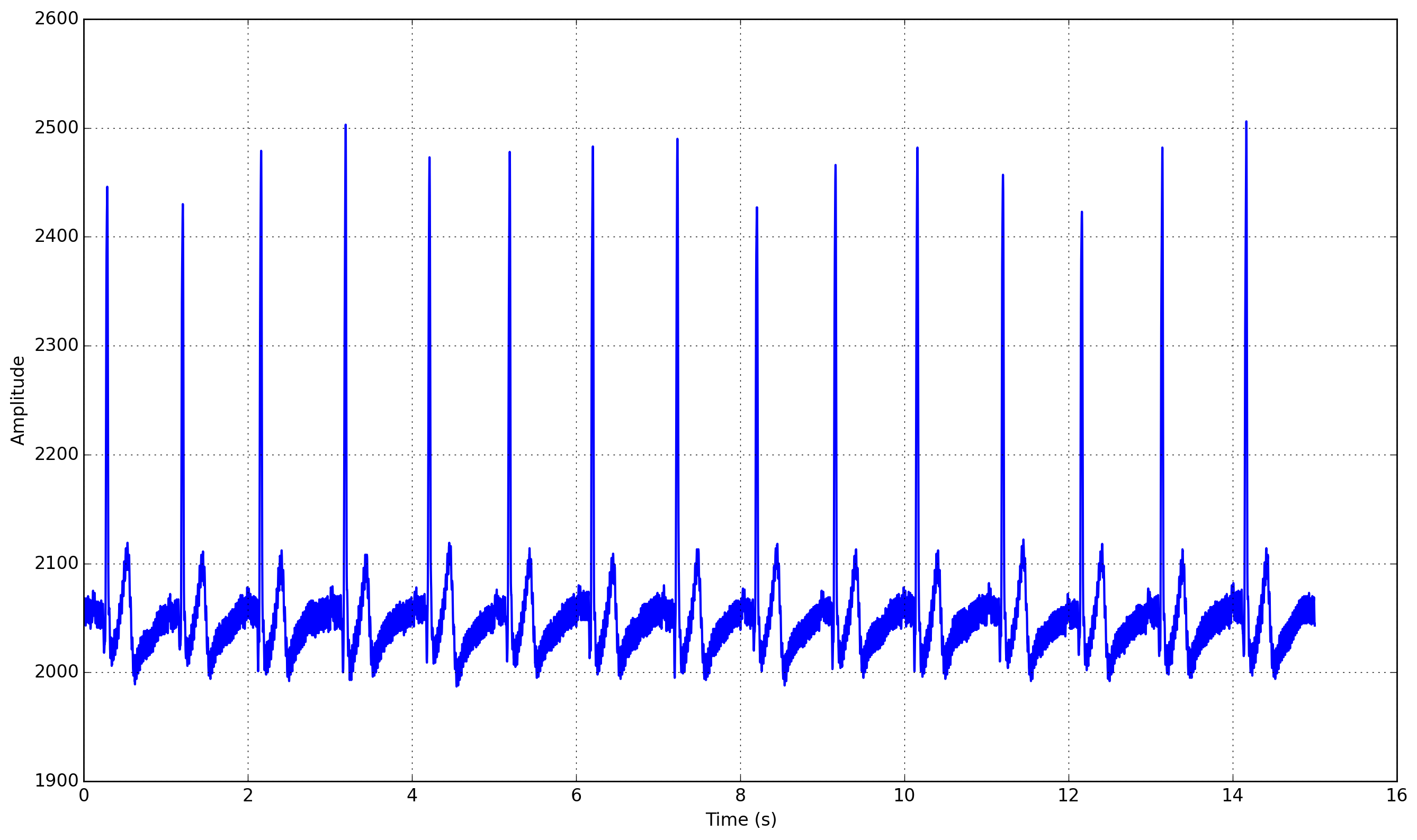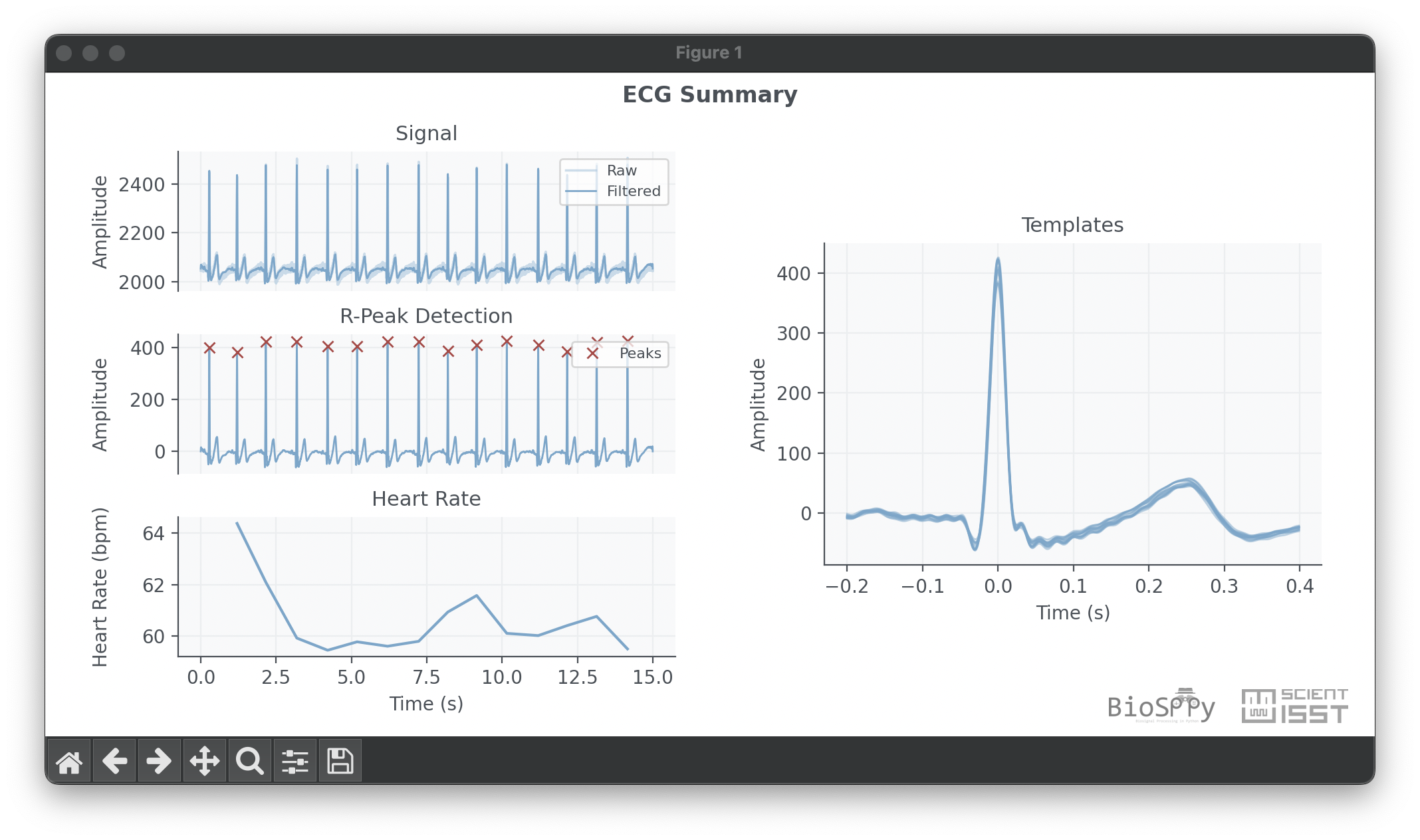# Tutorial¶

In this tutorial we will describe how biosppy enables the development of Pattern Recognition and Machine Learning workflows for the analysis of biosignals. The major goal of this package is to make these tools easily available to anyone wishing to start playing around with biosignal data, regardless of their level of knowledge in the field of Data Science. Throughout this tutorial we will discuss the major features of biosppy and introduce the terminology used by the package.

## What are Biosignals?¶

Biosignals, in the most general sense, are measurements of physical properties of biological systems. These include the measurement of properties at the cellular level, such as concentrations of molecules, membrane potentials, and DNA assays. On a higher level, for a group of specialized cells (i.e. an organ) we are able to measure properties such as cell counts and histology, organ secretions, and electrical activity (the electrical system of the heart, for instance). Finally, for complex biological systems like the human being, biosignals also include blood and urine test measurements, core body temperature, motion tracking signals, and imaging techniques such as CAT and MRI scans. However, the term biosignal is most often applied to bioelectrical, time-varying signals, such as the electrocardiogram.

The task of obtaining biosignals of good quality is time-consuming, and typically requires the use of costly hardware. Access to these instruments is, therefore, usually restricted to research institutes, medical centers, and hospitals. However, recent projects like BITalino or OpenBCI have lowered the entry barriers of biosignal acquisition, fostering the Do-It-Yourself and Maker communities to develop physiological computing applications. You can find a list of biosignal platform here.

The following sub-sections briefly describe the biosignals covered by biosppy.

### Blood Volume Pulse¶

Blood Volume Pulse (BVP) signals are…

### Electrocardiogram¶

Electrocardiogrm (ECG) signals are…

### Electrodermal Activity¶

Electrodermal Activity (EDA) signals are…

### Electroencephalogram¶

Electroencephalogram (EEG) signals are…

### Electromyogram¶

Electromyogram (EMG) signals are a measure of the electrical activity of muscles. There are two types of sensors that can be used to record this electrical activity, in particular surface EMG (sEMG), measured by non-invasive electrodes, and intramuscular EMG. Out of the two, sEMG allows for non-invasive electrodes to be applied at the body surface, that measure muscle activity. In sEMG, contact with the skin can be done with standard pre-gelled electrodes, dry Ag/AgCl electrodes or conductive textiles. Normally, there are three electrodes in an sEMG interface: two electrodes work on bipolar differential measurement and the other one is attached to a neutral zone, to serve as the reference point. After being recorded, this signal can be processed in time, frequency and time-frequency domains. In an EMG signal, when the muscle is in a relaxed state, this corresponds to the baseline activity. The bursts of activity match the muscular activations and have a random shape, meaning that a raw recording of contractions cannot be exactly reproduced. The onset of an event corresponds to the beginning of the burst.

### Respiration¶

Respiration (Resp) signals are…

To do.

## A Note on Return Objects¶

Before we dig into the core aspects of the package, you will quickly notice that many of the methods and functions defined here return a custom object class. This return class is defined in `biosppy.utils.ReturnTuple`. The goal of this return class is to strengthen the semantic relationship between a function’s output variables, their names, and what is described in the documentation. Consider the following function definition:

```def compute(a, b):
"""Simultaneously compute the sum, subtraction, multiplication and
division between two integers.

Args:
a (int): First input integer.
b (int): Second input integer.

Returns:
(tuple): containing:
sum (int): Sum (a + b).
sub (int): Subtraction (a - b).
mult (int): Multiplication (a * b).
div (int): Integer division (a / b).

"""

if b == 0:
raise ValueError("Input 'b' cannot be zero.")

v1 = a + b
v2 = a - b
v3 = a * b
v4 = a / b

return v1, v2, v3, v4
```

Note that Python doesn’t actually support returning multiple objects. In this case, the `return` statement packs the objects into a tuple.

```>>> out = compute(4, 50)
>>> type(out)
<type 'tuple'>
>>> print out
(54, -46, 200, 0)
```

This is pretty straightforward, yet it shows one disadvantage of the native Python return pattern: the semantics of the output elements (i.e. what each variable actually represents) are only implicitly defined with the ordering of the docstring. If there isn’t a dosctring available (yikes!), the only way to figure out the meaning of the output is by analyzing the code itself.

This is not necessarily a bad thing. One should always try to understand, at least in broad terms, how any given function works. However, the initial steps of the data analysis process encompass a lot of experimentation and interactive exploration of the data. This is important in order to have an initial sense of the quality of the data and what information we may be able to extract. In this case, the user typically already knows what a function does, but it is cumbersome to remember by heart the order of the outputs, without having to constantly check out the documentation.

For instance, does the numpy.histogram function first return the edges or the values of the histogram? Maybe it’s the edges first, which correspond to the x axis. Oops, it’s actually the other way around…

In this case, it could be useful to have an explicit reference directly in the return object to what each variable represents. Returning to the example above, we would like to have something like:

```>>> out = compute(4, 50)
>>> print out
(sum=54, sub=-46, mult=200, div=0)
```

This is exactly what `biosppy.utils.ReturnTuple` accomplishes. Rewriting the compute function to work with ReturnTuple is simple. Just construct the return object with a tuple of strings with names for each output variable:

```from biosppy import utils

def compute_new(a, b):
"""Simultaneously compute the sum, subtraction, multiplication and
division between two integers.

Args:
a (int): First input integer.
b (int): Second input integer.

Returns:
(ReturnTuple): containing:
sum (int): Sum (a + b).
sub (int): Subtraction (a - b).
mult (int): Multiplication (a * b).
div (int): Integer division (a / b).

"""

if b == 0:
raise ValueError("Input 'b' cannot be zero.")

v1 = a + b
v2 = a - b
v3 = a * b
v4 = a / b

# build the return object
output = utils.ReturnTuple((v1, v2, v3, v4), ('sum', 'sub', 'mult', 'div'))

return output
```

The output now becomes:

```>>> out = compute_new(4, 50)
>>> print out
ReturnTuple(sum=54, sub=-46, mult=200, div=0)
```

It allows to access a specific variable by key, like a dictionary:

```>>> out['sum']
54
```

And to list all the available keys:

```>>> out.keys()
['sum', 'sub', 'mult', 'div']
```

It is also possible to convert the object to a more traditional dictionary, specifically an OrderedDict:

```>>> d = out.as_dict()
>>> print d
OrderedDict([('sum', 54), ('sub', -46), ('mult', 200), ('div', 0)])
```

Dictionary-like unpacking is supported:

```>>> some_function(**out)
```

ReturnTuple is heavily inspired by namedtuple, but without the dynamic class generation at object creation. It is a subclass of tuple, therefore it maintains compatibility with the native return pattern. It is still possible to unpack the variables in the usual way:

```>>> a, b, c, d = compute_new(4, 50)
>>> print a, b, c, d
54 -46 200 0
```

The behavior is slightly different when only one variable is returned. In this case it is necessary to explicitly unpack a one-element tuple:

```from biosppy import utils

def foo():
"""Returns 'bar'."""

out = 'bar'

return utils.ReturnTuple((out, ), ('out', ))
```
```>>> out, = foo()
>>> print out
'bar'
```

## A First Approach¶

One of the major goals of biosppy is to provide an easy starting point into the world of biosignal processing. For that reason, we provide simple turnkey solutions for each of the supported biosignal types. These functions implement typical methods to filter, transform, and extract signal features. Let’s see how this works for the example of the ECG signal.

The GitHub repository includes a few example signals (see here). To load and plot the raw ECG signal follow:

```>>> import numpy as np
>>> import pylab as pl
>>> from biosppy import storage
>>>
>>> Fs = mdata['sampling_rate']
>>> N = len(signal)  # number of samples
>>> T = (N - 1) / Fs  # duration
>>> ts = np.linspace(0, T, N, endpoint=False)  # relative timestamps
>>> pl.plot(ts, signal, lw=2)
>>> pl.grid()
>>> pl.show()
```

This should produce a similar output to the one shown below.This signal is a Lead I ECG signal acquired at 1000 Hz, with a resolution of 12 bit. Although of good quality, it exhibits powerline noise interference, has a DC offset resulting from the acquisition device, and we can also observe the influence of breathing in the variability of R-peak amplitudes.

We can minimize the effects of these artifacts and extract a bunch of features with the `biosppy.signals.ecg.ecg` function:

```>>> from biosppy.signals import ecg
>>> out = ecg.ecg(signal=signal, sampling_rate=Fs, show=True)
```

It should produce a plot like the one below.To do..

To do..

To do..

To do..